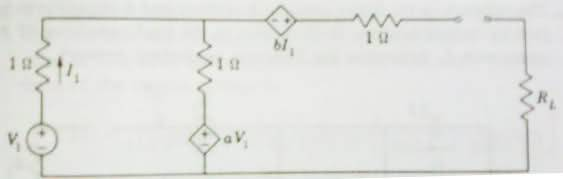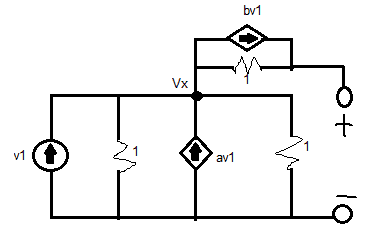# Thevenin equivalent circuit

• Engineering
I need help finding the thevenin equivalent voltage for the following network:i did source transformations so that i could analyze it using the node voltage method.my equations are:
I1 = v1
(a) vx + vx - vT = v1 + av1 - bv1
2vx - vT = v1 + av1 - bv1
(b) vT - vx + vT = bv1
2vT - vx = bv1

when i simplify (by substituting vx), i get
vT = v1/3(a + b + 1)

vT = v1/2(a + b + 1 - ab)

Last edited:

I need help finding the thevenin equivalent voltage for the following network:i did source transformations so that i could analyze it using the node voltage method.my equations are:
I1 = v1
(a) vx + vx - vT = v1 + av1 - bv1
2vx - vT = v1 + av1 - bv1
(b) vT - vx + vT = bv1
2vT - vx = bv1

when i simplify (by substituting vx), i get
vT = v1/3(a + b + 1)

vT = v1/2(a + b + 1 - ab)

I think I've spotted a problem with how you've redrawn your circuit.

I think it be should more like this,EDIT: There's really no reason to apply source transformations to the circuit at all, the original circuit can be solve by writing two KVL equations.

$$\text{Equation 1:}$$

$$Voc = I_{1} + aV_{1} + bI_{1}$$

$$\text{Equation 2:}$$

$$-V_{1} + 2I_{1} + aV_{1} = 0$$

$$\text{Equation 2} \Rightarrow \text{Equation 1}$$

This gives the expected result.

The Latex doesn't seem to be working, but hopefully you can follow through my work.

#### Attachments

Last edited:

can you explain for first KVL equation? i'm not sure where the I1 comes from

can you explain for first KVL equation? i'm not sure where the I1 comes from

Here we are writing a KVL in the righthand loop.

Notice that the loop is open thus there is no current flowing in this loop except for the current in the vertical, leftmost branch.

This current is clearly I1 by writing a KCL at the top node.

Clear?

Here we are writing a KVL in the righthand loop.

Notice that the loop is open thus there is no current flowing in this loop except for the current in the vertical, leftmost branch.

This current is clearly I1 by writing a KCL at the top node.

Clear?

yup. thanks.